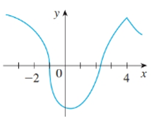# To state: The number of points where given graph of function f is not differentiable.### Single Variable Calculus: Concepts...

4th Edition
James Stewart
Publisher: Cengage Learning
ISBN: 9781337687805### Single Variable Calculus: Concepts...

4th Edition
James Stewart
Publisher: Cengage Learning
ISBN: 9781337687805

#### Solutions

Chapter 2.7, Problem 37E
To determine

## To state:The number of points where given graph of function f is not differentiable.

Expert Solution

The numbers are -1 and 4 where f is not differentiable.

### Explanation of Solution

Given: The graph of function.

Let f be any function and graph of this function is given.

It has to find numbers of points at which f is not differentiable.The function f is not differentiable at the following numbers:

Because the tangent is vertical at x=1 .

x=4 Because there is a corner in graph of f .

Hence, the numbers are -1 and 4 where f is not differentiable.

### Have a homework question?

Subscribe to bartleby learn! Ask subject matter experts 30 homework questions each month. Plus, you’ll have access to millions of step-by-step textbook answers!